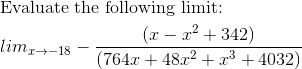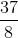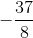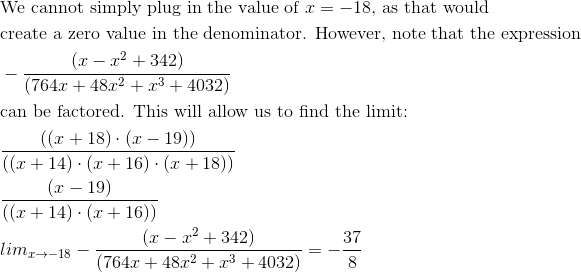Calculus 3 : Limits

Example Questions

← Previous 1 3 4 5 6 7 8 9 35 36

Example Question #1 : Limits

Evaluate: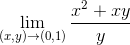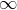Does not exist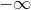Explanation:

Since we won't have any divided by zero problems, we can just evaluate at the point.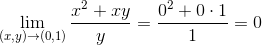Example Question #1 : Partial Derivatives

Calculate the following limit.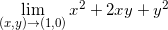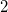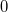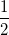Explanation:

We can calculate the limitby simply plugging in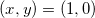because the value is defined at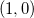and around that point.

So we get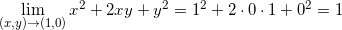.

Example Question #3 : Limits

Calculate the following limit.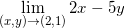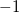Explanation:

We can calculate the limitby simply plugging in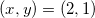because the value is defined at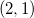and at every value in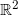.

So we get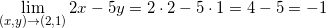.

Example Question #4 : Limits

Evaluate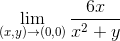Does not existDoes not exist

Explanation:

If we try evaluating the limit along two different paths,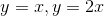, we have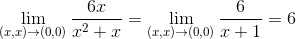And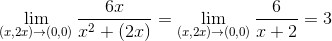Since evaluating along these two paths yielded diffferent values, the limit does not exist.

Example Question #5 : Limits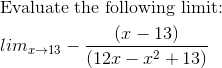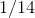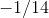Explanation: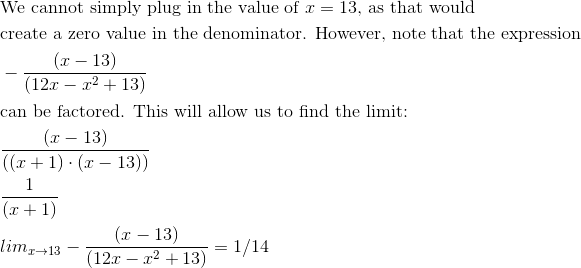Example Question #6 : Limits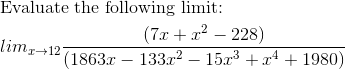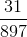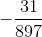Explanation: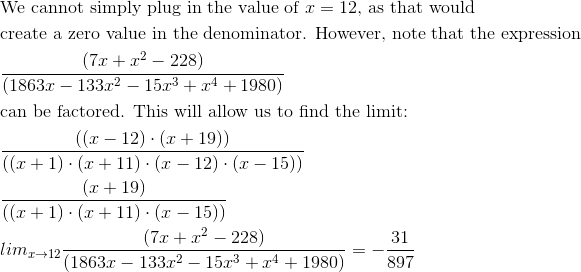Example Question #7 : Limits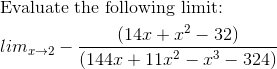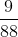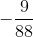Explanation: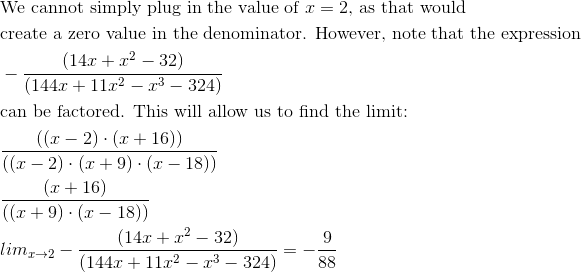Example Question #8 : Limits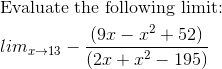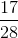Explanation: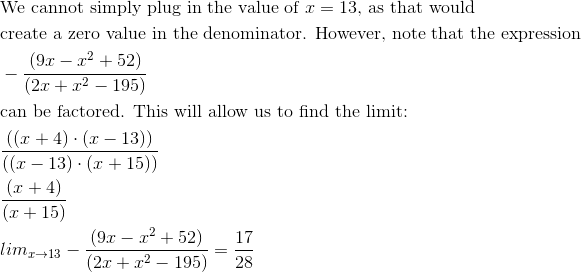Example Question #9 : Limits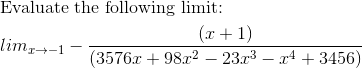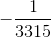Explanation: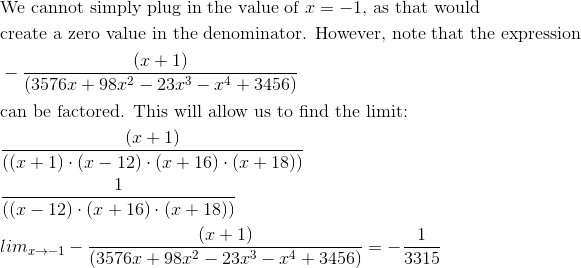Example Question #10 : Limits